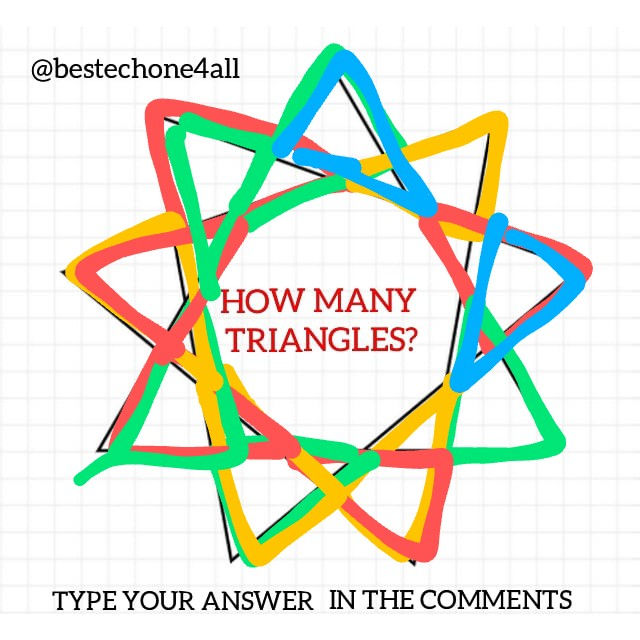top of page

# How many Triangles are there?

Can you find the exact number of triangles in this image?It seems complex but it can be broken down into three simple steps as follows:

Step 1: Count the Large triangles

This one is the easiest. It will give 3 triangles. A common mistake here will be to count each vertex as a new triangle thus giving the wrong answer 9. Here's an illustration:Step 2: Count all the medium triangles.

This is the most complex part. You need to notice here that each vertex has 2 medium triangles and you simply multiply the number of vertices (9) by 2 to get 18 triangles.Or you could count all of them one by one the old fashioned way as follows:A little messy yes but you get the point.

Step 3: Count the small triangles.

This will give 9 triangles as follows:Lastly, you should also count the letter A(3) and the number 4(1) since they include triangles.

This gives a total of : 3+18+9+4=34 triangles.

Did you get it?? Share to challenge your friends.